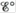# The amount of manganese in steel is determined by changing it to permanganate ion. The steel is first dissolved in nitric acid, producing Mn 2+ ions. These ions are then oxidized to the deeply colored MnO 4 − ions by periodate ion (IO 4 − ) in acid solution. a. Complete and balance an equation describing each of the above reactions. b. Calculate and Δ G° at 25°C for each reaction.### Chemistry: An Atoms First Approach

2nd Edition
Steven S. Zumdahl + 1 other
Publisher: Cengage Learning
ISBN: 9781305079243

#### Solutions

Chapter
Section### Chemistry: An Atoms First Approach

2nd Edition
Steven S. Zumdahl + 1 other
Publisher: Cengage Learning
ISBN: 9781305079243
Chapter 17, Problem 50E
Textbook Problem
1 views

## The amount of manganese in steel is determined by changing it to permanganate ion. The steel is first dissolved in nitric acid, producing Mn2+ ions. These ions are then oxidized to the deeply colored MnO4− ions by periodate ion (IO4−) in acid solution.a. Complete and balance an equation describing each of the above reactions.b. Calculateand ΔG° at 25°C for each reaction.

(a)

Interpretation Introduction

Interpretation: The complete and balanced given chemical reaction corresponding to the stated reaction is to be determined. The standard cell potential Eο and standard Gibbs free energy ΔGο at 2οC are to be calculated.

Concept introduction: The chemical equation is said to be balanced when the number of atoms of chemical species on the reactant side is equal to the number of chemical species on the product side. Cell potential is defined as the measure of energy per unit charge available from the redox reaction to carry out the reaction. Gibbs free energy is a thermodynamic quantity that is used to calculate the maximum work of reversible reaction performed by a system. It is equal to the difference between the enthalpy and the product of entropy at absolute temperature.

To determine: The complete and balanced given chemical reaction.

### Explanation of Solution

Explanation

The balanced chemical reaction representing the dissolution of steel in nitric acid to produce Mn2+ is as follows,

3Mn(s)+8H+(aq)+2NO3-(aq)3Mn2+(aq)+2NO(g)+4H2O(l) (1)

The balanced chemical reaction representing the oxidation of Mn2+ ions into deeply colored

(b)

Interpretation Introduction

Interpretation: The complete and balanced given chemical reaction corresponding to the stated reaction is to be determined. The standard cell potential Eο and standard Gibbs free energy ΔGο at 2οC are to be calculated.

Concept introduction: The chemical equation is said to be balanced when the number of atoms of chemical species on the reactant side is equal to the number of chemical species on the product side. Cell potential is defined as the measure of energy per unit charge available from the redox reaction to carry out the reaction. Gibbs free energy is a thermodynamic quantity that is used to calculate the maximum work of reversible reaction performed by a system. It is equal to the difference between the enthalpy and the product of entropy at absolute temperature.

To determine: The standard cell potential Eο and standard Gibbs free energy ΔGο at 2οC .

### Still sussing out bartleby?

Check out a sample textbook solution.

See a sample solution

#### The Solution to Your Study Problems

Bartleby provides explanations to thousands of textbook problems written by our experts, many with advanced degrees!

Get Started

Find more solutions based on key concepts
A ________ is dissolved in a solvent. a. molecule b. salt c. solute d. chemical bond

Biology: The Unity and Diversity of Life (MindTap Course List)

What elements drive a nuclear fusion reaction?

Fundamentals of Physical Geography

Added sugars can be useful in meeting the high carbohydrate needs of some athletes. T F

Nutrition: Concepts and Controversies - Standalone book (MindTap Course List)

Explain the concept of a mole in your own words.

Chemistry for Engineering Students

What physical property is characteristic of optically active molecules?

Chemistry for Today: General, Organic, and Biochemistry

What is a joint?

Human Biology (MindTap Course List)

A piece of insulated wire is shaped into a figure eight as shown in Figure P.SI.20. For simplicity, model the t...

Physics for Scientists and Engineers, Technology Update (No access codes included)

Which is older, the Moon or the Sun? How do you know?

Foundations of Astronomy (MindTap Course List)

What are abyssal plains? What is unique about them?

Oceanography: An Invitation To Marine Science, Loose-leaf Versin